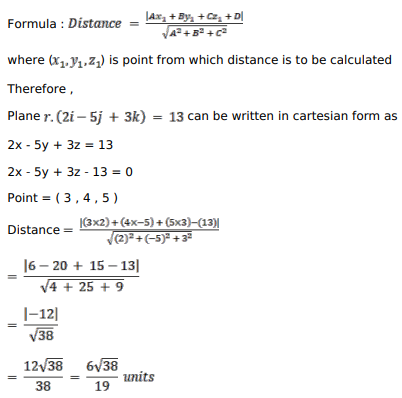# Solve this followingQuestion:

Find the distance of the point $(3,4,5)$ from the plane $\overrightarrow{\mathrm{r}} \cdot(2 \hat{\mathrm{i}}-5 \hat{\mathrm{j}}+3 \hat{\mathrm{k}})=13$.

Solution: